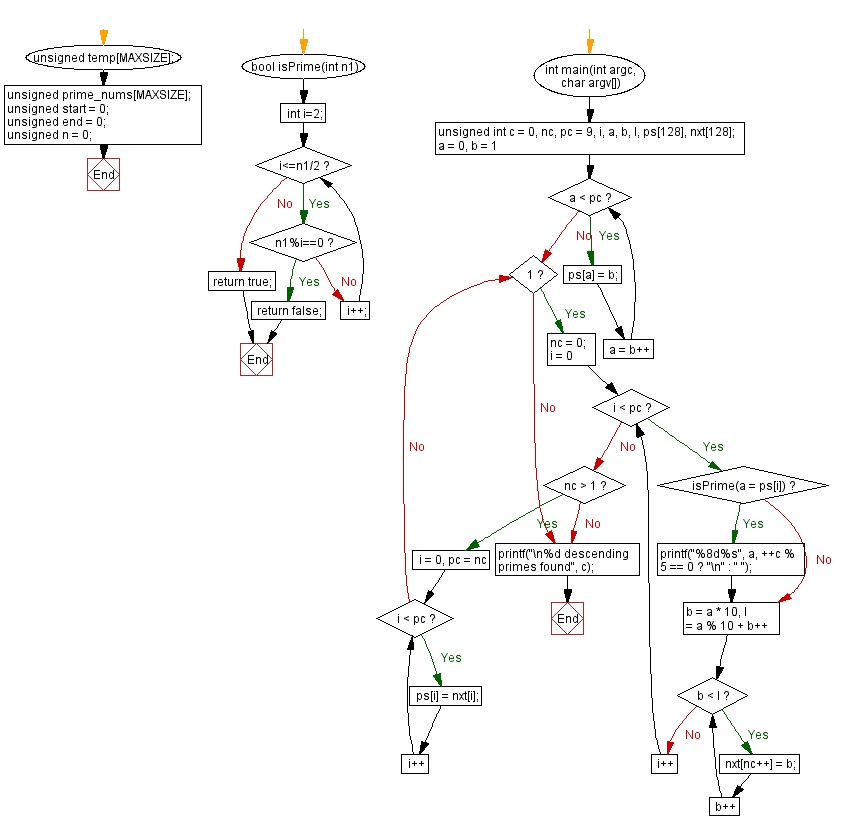﻿ C Program - Prime number in strictly descending decimal digit order# C Exercises: Prime number in strictly descending decimal digit order

## C Programming Challenges: Exercise-32 with Solution

Write a C# Sharp program to create and display all prime numbers in strictly descending decimal digit order.

Sample Data:
2, 3, 5, 7, 31, 41, 43, 53, 61, 71, 73, 83, 97, 421, 431.....

C Code:

``````//Source:shorturl.at/eFRX1
#include <stdio.h>
#include <stdlib.h>
#include <stdbool.h>
#include <math.h>

#define MAXSIZE 1000

unsigned temp[MAXSIZE];
unsigned prime_nums[MAXSIZE];

unsigned start = 0;
unsigned end = 0;
unsigned n = 0;

bool isPrime(int n1)
{
int i=2;
while(i<=n1/2)
{
if(n1%i==0)
return false;
else
i++;
}
return true;
}

int main(int argc, char argv[])
{
unsigned int c = 0, nc, pc = 9, i, a, b, l,
ps, nxt;
for (a = 0, b = 1; a < pc; a = b++) ps[a] = b;
while (1) {
nc = 0;
for (i = 0; i < pc; i++) {
if (isPrime(a = ps[i]))
printf("%8d%s", a, ++c % 5 == 0 ? "\n" : " ");
for (b = a * 10, l = a % 10 + b++; b < l; b++)
nxt[nc++] = b;
}
if (nc > 1) for(i = 0, pc = nc; i < pc; i++) ps[i] = nxt[i];
else break;
}
printf("\n%d descending primes found", c);
}
``````

Sample Output:

```       1        2        3        5        7
31       41       43       53       61
71       73       83       97      421
431      521      541      631      641
643      653      743      751      761
821      853      863      941      953
971      983     5431     6421     6521
7321     7541     7621     7643     8431
8521     8543     8641     8731     8741
8753     8761     9421     9431     9521
9631     9643     9721     9743     9851
9871    75431    76421    76541    76543
86531    87421    87541    87631    87641
87643    94321    96431    97651    98321
98543    98621    98641    98731   764321
865321   876431   975421   986543   987541
987631  8764321  8765321  9754321  9875321
97654321 98764321 98765431
88 descending primes found
```

Flowchart:## C Programming Code Editor:

Contribute your code and comments through Disqus.

Previous C Programming Exercise: Arithmetic and geometric progression series.
Next C Programming Exercise: Attractive numbers up to 100.

What is the difficulty level of this exercise?

Test your Programming skills with w3resource's quiz.

﻿